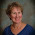## Monday, October 11, 2010

### Casting Out Nines

Did you know that the digits of any multiple of 9 add up to a multiple of 9?* For example, 9 * 125 = 1125, and 1+1+2+5 = 9.  And that's the basis for lots of number tricks.

Here's a version of an online trick using a crystal ball to predict your number.

But some of the tricksters aren't careful enough about their math, and can mess up. Shecky, at Math Frolic, pointed to this one (get your calculator out), that tells you your original number, and the digits you chose later in the process. But it messed up for me. I think it will mess up about one-tenth of the time, actually.

Here's how it goes:

On a PIECE of PAPER, write a number between 10 and 10,000.
I chose 13.
Multiply it by 4.
52
Now add 5 to the result.
57
Now multiply the result by 75.
4275
Now choose any TWO digits from the result and add them to the result.
Example: 591673 + 69
I chose the 5 and 2, and added 52.
Now multiply the result by 3.
Lets multiply the result by 3 again to make it more difficult.
I multiplied by 9 to get 38943.
Now substitute one digit with an "X" and enter your result below.
I chose the 9.

The first number was: 12
Then you choose: 52
And you substituted the number 0 with an "X"

If I went back one step and put the x in for the 8 instead, it got everything right.

What went wrong?

By the way, casting out nines refers to a way to check your addition (important back when we didn't use calculators and computers). It depends on the fact that the remainder after division by nine is always the same for a number and the sum of its digits.

______
*Why?

1.I read this and thought it was really neat and related to what we have been talking about in my college math class. I also tried it with the number 15 and it took me a couple of times to get it but finally I got it. Its a very neat math trick!

2.Glad you liked it. I'm curious what math class and topic this related to.

3.So you can also check multiplication with casting out nines. You have to multiply the check digits and then compare to the check digit of the answer.

4.Your observation, casting out nines, and the trick you show, are all consequences of using a base-10 number system. Since 10 divided by 9 has a remainder of 1, multiplying by any power of 10 is an identity operation, as far as divisibility by 9 goes. This means that you can add the digits together, and the result will give the same remainder when divided by 9 as the original, since the powers of 10 implied by our place notation system are all the same as 1. If the result is greater than 9, you can repeat the process until you arrive at a single digit (AKA the digital root). This will be the remainder when the original was divided by 9, except you will get 9 instead of 0 if the (non-zero) number was divisible by 9. Which is why your trick will fail when you replace a 9 with X.

If we call your original number A and the two digit number you add later B, the result before you multiply by 9 will be 300A + 375 + B. Since your final result is guaranteed to be divisible by 9, casting out nines will give a result of 9. The number replaced by X can then be found by casting out nines for the remaining digits, and subtracting that value from 9. You then replace the X with that result to reconstitute the final number. Dividing by 9 and subtracting 375 leaves you with 300A + B. Since 300A must end in 00, the last two digits are B. Subtract those, then divide by 300 to get the original number A.

The weakness of this trick is that it can't distinguish a 0 from a 9 as X. Your example has a digital root of 9 showing, so the program reconstructed the number (incorrectly) as 38043, and backtracked to find 12 instead of 13.

Unknown Correspondent